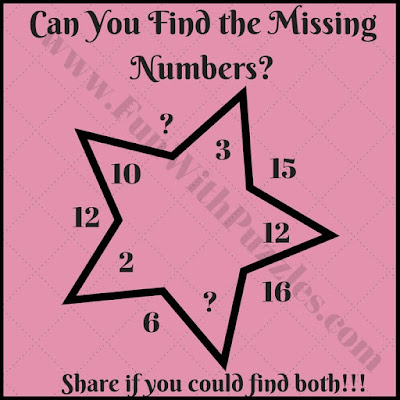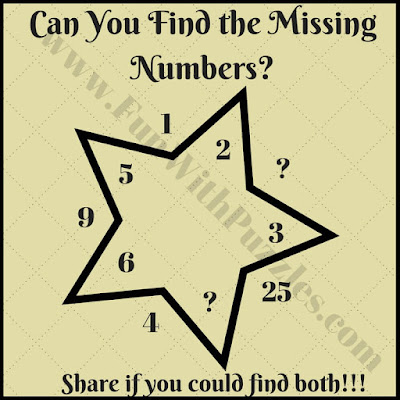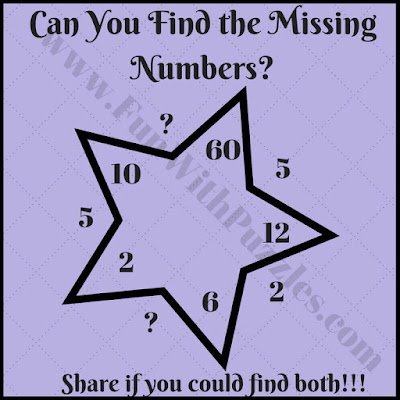We have been posting Circle Maths Puzzles and Brain Teasers and these puzzles are very much liked by our audience. In these some numbers are given around the circle which follows some mathematical pattern or has some mathematical relationship with each other. One has to find this relationship and then solve it for the missing number. Here we have a similar math brain teaser in which we have changed the shape of the picture from a circle to a star.
In these Star Math Brain Teasers, there are some numbers in and outside the star. These numbers have some mathematical relationship with each other. One has to find this relationship and then solve it for the missing numbers.
So are you ready to find the missing numbers in these puzzles? These Star Math Puzzles are very easy puzzles. We will post some tougher Star math brain teasers next time. Do write your answers in the comment section of this post.1. Simple math star picture puzzle question for Kids2. Easy math star picture puzzle question for Kids3. Quick math star picture riddle for Kids4. Math star picture brain teaser for Kids5. Interesting Fun Star Math Brain Teaser for Kids